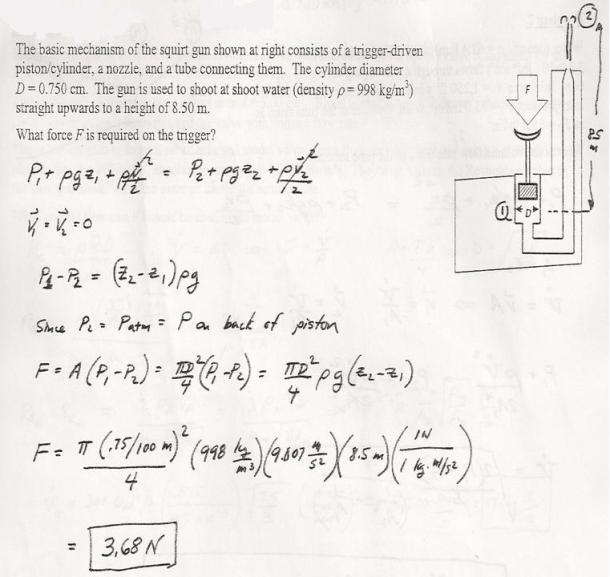fluid mechanics math problems engineering equations fluid mechanics basic mechanics school homework engineering math fluids formulas fluid mechanics problems fluid mechanics problem solutions to fluids problems full solution fluid mechanics engineering fluids problem solution fluid mechanics math problems engineering equations fluid mechanics basic mechanics school homework engineering math fluid mechanics formulas fluid problems fluid mechanics problem solutions to fluid mechanics problems full solution fluid mechanics engineering fluid mechanics problem solution
fluid mechanics math problems engineering equations fluid mechanics basic mechanics school homework engineering math fluids formulas fluid mechanics problems fluid mechanics problem solutions to fluids problems full solution fluid mechanics engineering fluids problem solution fluid mechanics math problems engineering equations fluid mechanics basic mechanics school homework engineering math fluid mechanics formulas fluid problems fluid mechanics problem solutions to fluid mechanics problems full solution fluid mechanics engineering fluid mechanics problem solution
Highalphabet Home Page fluid mechanics solutions fluids math solved Fluid Mechanics Page 1
The basic mechanism of the squirt gun shown at right consists of a trigger-driven piston/cylinder, a nozzle, and a tube connecting them. The cylinder diameter D=0.75 cm. The gun is used to shoot water (density p=998 kg/m^3) straight upwards to a height of 8.5 m. What force F is required on the trigger?The basic mechanism of the squirt gun shown at right consists of a trigger-driven piston/cylinder, a nozzle, and a tube connecting them. The cylinder diameter D=0.75 cm. The gun is used to shoot water (density p=998 kg/m^3) straight upwards to a height of 8.5 m. What force F is required on the trigger?